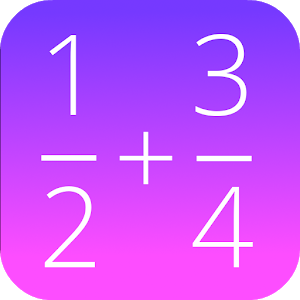Italiano

Ogni giorno offriamo licenze per app e giochi Android che altrimenti dovresti acquistare.\$0.99
gratis oggi

# Android Giveaway of the Day - Fractions Math Pro

This math app is a fractions calculator with various functions!
\$0.99 gratis oggi
User Rating: 5 (100%) 0 (0%)

Fractions Math Pro
è disponibile come Giveaway of the day!L’offerta per scaricarlo e installarlo è limitata.This calculator is the pro version of 'Fractions Math', and completely without advertisement.

This math app is a fractions calculator with various functions:

- add, subtract, multiply and divide fractions.
- convert fractions in decimals,
- simplify fractions
- compare fractions
- find the lowest common denominator
- fraction trainer, to learn fraction calculations

GK Apps

Education

4.0

279k

Everyone

1.6 and up

## Commenti su Fractions Math Pro

Thank you for voting!